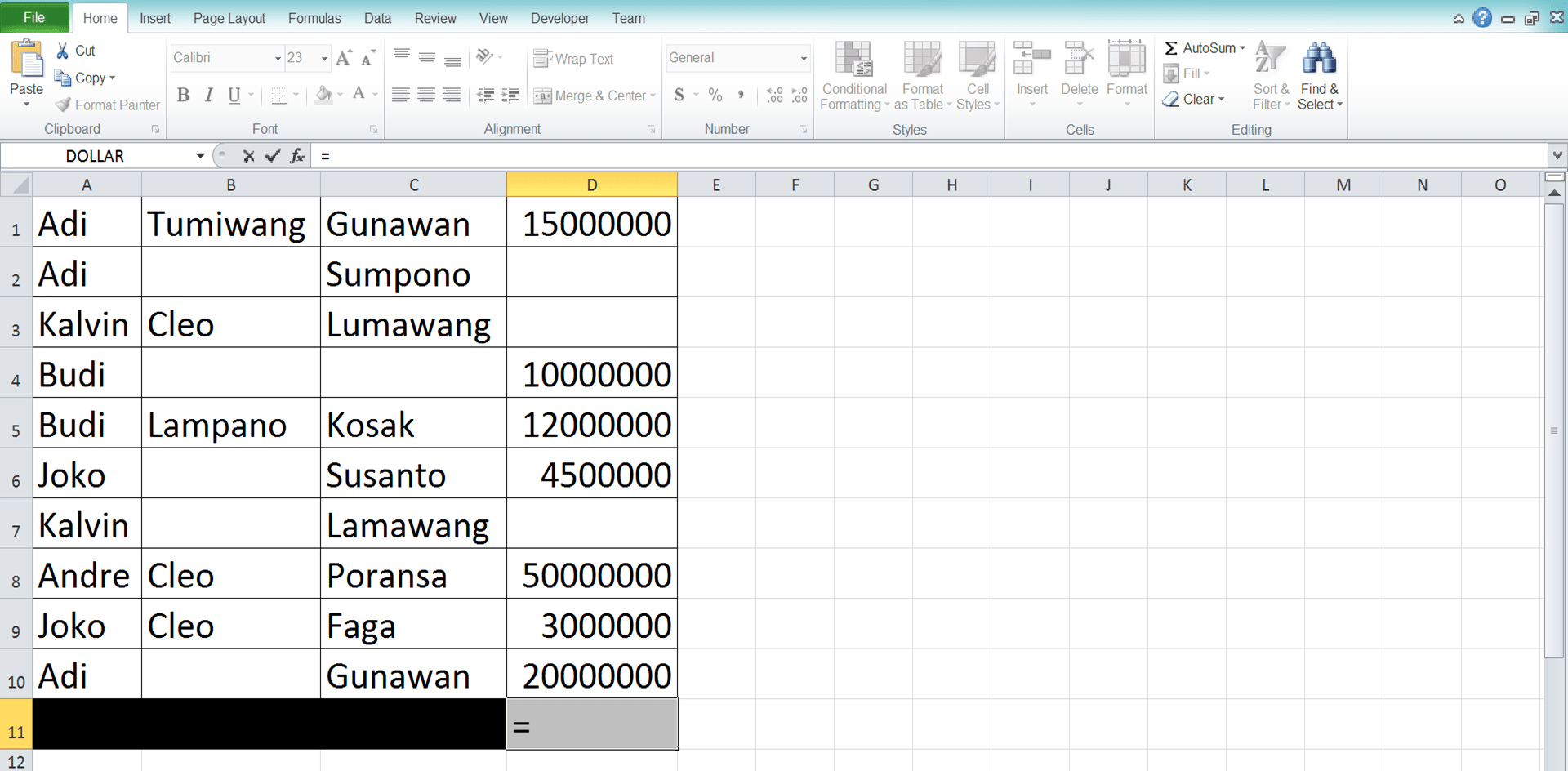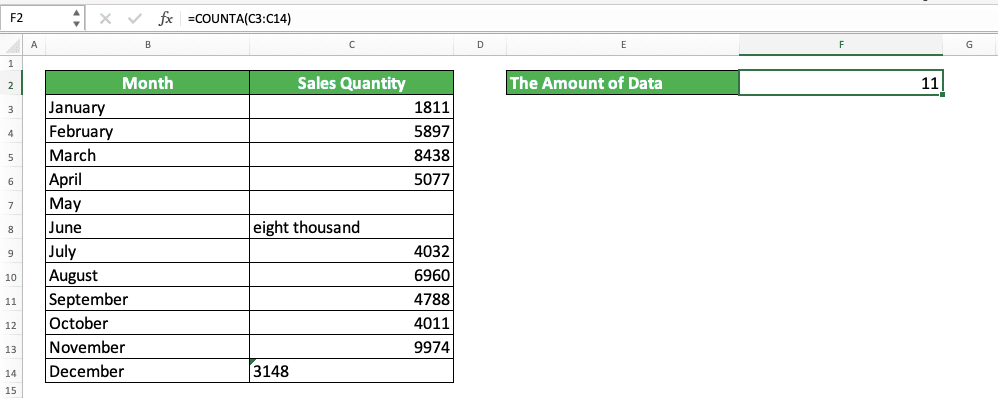Using COUNT Function in Excel: Usabilities, Example, and Writing Steps - Compute Expert

# Using COUNT Function in Excel:Usabilities, Example, and Writing Steps

Home >> Excel Tutorials from Compute Expert >> Excel Formulas List >> Using COUNT Function in Excel: Usabilities, Example, and Writing Steps

In this tutorial, we will discuss how to use the COUNT function in excel completely. If you want to count how many numbers you have, then COUNT is the formula to help you do that fast.

Disclaimer: This post may contain affiliate links from which we earn commission from qualifying purchases/actions at no additional cost for you. Learn more

## COUNT Usabilities

We can use COUNT to count the number of numbers in excel.

## COUNT Result

The result which COUNT gives is a number that represents how many number data you have.

## Excel Version in Which COUNT can Start to be Used

COUNT can start to be used by excel users since excel 2003.

## How to Write It and Its Inputs

Generally, the writing form of COUNT can be illustrated like the following.

=COUNT(value_1, value_2, …)

value_1, value_2,… are the data you want to count the numbers from. You can type your data directly/input cell coordinates/ranges here up to 255 different inputs. However, usually, we only input one cell range when we write a COUNT formula.

## Example of Usage and Result

To understand more about COUNT, here is its implementation and result example in excel.In the example, we can see how the COUNT writing is done and its result. This formula only counts the number type of data in its cell range input. Other data types like empty cells, text, or text-type numbers will be ignored.

In this example, we can also see the most often input form given for this formula, which is a cell range. COUNT can also accept other input forms like directly typed data and cell coordinates. However, we mostly give a cell range as its input.

Don’t forget, if you give more than one input, then you need to type comma signs between the inputs (like in the general writing form given in the previous part).

## Writing Steps

The following will explain the detailed steps to write the COUNT formula in excel. In this formula, we only need to give one type of input, which is cell ranges/data/cell coordinates.

1. Type an equal sign ( = ) in the cell where you want to put the number counting result2. Type COUNT (can be with large and small letters) and an open bracket sign after =3. Input the cell range/data/cell coordinate from which you want to count your numbers. If you give more than one inputs, then give comma signs ( , ) between your inputs4. Type a close bracket sign after you have inputted all your inputs5. Press Enter
6. Done!## How to Count Data in Excel (Not Only Numbers): COUNTA

This is sometimes wrongly perceived by excel users. We think that COUNT can help us count the amount of all our data, where it actually can only count numbers.

If we want to count all data, not just numbers, then what is the formula we should use? COUNTA is the answer!

Generally, the writing of COUNTA is similar to COUNT as you can see below.

=COUNTA(value_1, value_2, …)

The input of COUNTA can also be the data typed directly in it, a cell coordinate, or a cell range. But, like COUNT, usually, we input one cell range as its input. If we give more than one input, then COUNTA can accept up to 255 different inputs.

To know better the difference between COUNTA and COUNT in counting data, here is a COUNTA implementation and result example.As you can see in the example above, COUNTA gives a different result from COUNT, using the same cell range input. COUNTA also counts text and numbers with text data type in the cell range.

As long as there is data in the cell or input unit, then COUNTA will count it too. This makes it a great formula if you want to count all data, whatever the type is, in your cell range!

If you want to learn more about COUNTA, you can visit this tutorial which discusses it in detail.

## Exercise

After you learn how to use COUNT, you can practice your understanding by doing the following exercise!

### Questions

1. How many numbers are in the table on the right?
2. How many numbers on column 6
3. How many numbers on row 3?

• COUNT will count all number data type in excel. That means it will also count dates, time, and percentages
• COUNT ignores TRUE and FALSE logic values, although TRUE and FALSE can be considered as 1 and 0 too in excel

Related tutorials you should learn:

Get updated excel info from Compute Expert by registering your email. It's free!